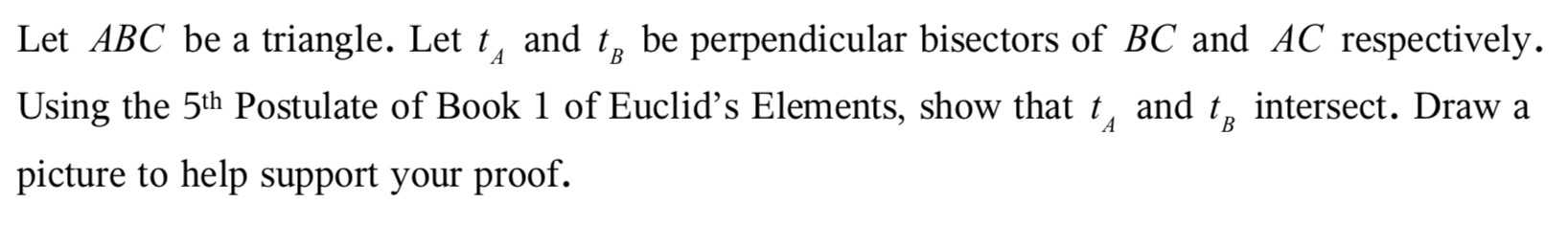# Let ABC be a triangle. Let t, and tg be perpendicular bisectors of BC and AC respectively.AВUsing the 5th Postulate of Book 1 of Euclid's Elements, show that t, and t, intersect. Draw aABpicture to help support your proof.

Question
76 viewshelp_outlineImage TranscriptioncloseLet ABC be a triangle. Let t, and tg be perpendicular bisectors of BC and AC respectively. A В Using the 5th Postulate of Book 1 of Euclid's Elements, show that t, and t, intersect. Draw a A B picture to help support your proof. fullscreen
check_circle

Step 1

Draw a triangle ABC and its two perpendicular bisectors in following,

Step 2

5th Postulate of Euclid’s elements is that if a straight falling on two straight lines makes the interior angles on the same side less than two right angles, the two straight lines, if produced indefinitely, meet on that side on which are the angles less than the two right angles.

Step 3

According to this Postulate, angle DBA and ang...

### Want to see the full answer?

See Solution

#### Want to see this answer and more?

Solutions are written by subject experts who are available 24/7. Questions are typically answered within 1 hour.*

See Solution
*Response times may vary by subject and question.
Tagged in

### Math